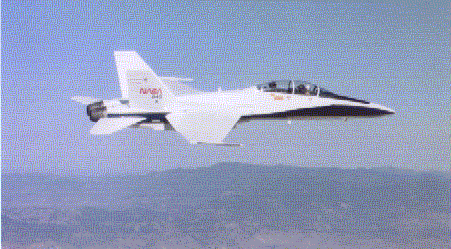+ Text Only Site
+ Non-Flash Version
+ Contact GlennThe Lift Equation Answers Identify each letter in the lift equation and list acceptable units for each quantity.  L = lift, newtons   Cl = lift coefficient, none   rho = density, kg/m3   V = velocity, m/s   A = area, m2    Use Wikipedia (http://en.wikipedia.org/wiki/Boeing_747), or a search engine, and look up the Boeing 747. What is its wing area? 511.0 m2 (the following numbers may vary slightly due to model number)   If the Boeing 747 above is cruising at 940 km/h, the density of the air is 1.20 g/L, and the lift coefficient is 1, what is the lift ? 20 900 000 newtons   Use Wikipedia (http://en.wikipedia.org/wiki/Boeing_777), or a search engine, to find the Boeing 777. What is its wing area? 377.7 m2   If the Boeing 777 is cruising at the same velocity under the same conditions as the 747 above, what is its lift? 15 400 000 newtons Look up the DC-8 on Wikipedia (http://en.wikipedia.org/wiki/DC-8), or use a search engine. What is its wing area? 267.9 m2   What is the DC-8's cruising velocity? 270 km/h   If the air density is 1.26 g/L and the lift coefficient is 1.5, what is the lift of the DC-8? 1 320 000 newtons   Find the F/A-18A Hornet on Wikipedia (http://en.wikipedia.org/wiki/F-18), or use a search engine. What is its wing area? 37.2 m2What is the F/A-18A's lift while it flies at 700 mph, the air density is 1.31 g/L, and the lift coefficient is 1.8? 4 610 000 newtons

Related Pages:
Standards
Activity
Worksheet
Lesson Index
Aerodynamics Index+ Inspector General Hotline + Equal Employment Opportunity Data Posted Pursuant to the No Fear Act + Budgets, Strategic Plans and Accountability Reports + Freedom of Information Act + The President's Management Agenda + NASA Privacy Statement, Disclaimer, and Accessibility CertificationEditor: Tom Benson NASA Official: Tom Benson Last Updated: Thu, May 13 02:38:26 PM EDT 2021 + Contact Glenn